Math Skills: How to Calculate, Convert, and Use Percents

 What is Percent?

If you listen to the radio, watch the television, or read a newspaper you can't help but hear or see phrases like "25% off today only" or "all merchandise 10% off" or "going out of business mark-downs of 50%." What does all this mean?

Percent means "parts per hundred." What you need to do is think of one whole as being divided into 100 parts. If you have all 100 of those parts, you have 100%. Notice the symbol we use for percent (%). If you have only 95 of the parts, you have 95%.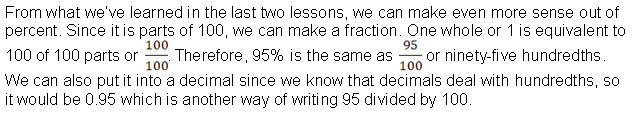Converting Between Decimals and Percents

To turn a number (either an integer or a decimal) into a percent, simply multiply by 100. That is the same as moving the decimal point two places to the right. You may need to round to the desired precision. Add a percent (%) sign.

0.32 as a percent is 32%

38.59 = 3859%

0.002 = 0.2%

To turn a percent into an integer or decimal number, simply divide by 100. That is the same as moving the decimal point two places to the left. Take off the percent (%) sign.

50% as a decimal is 0.50

3.5% = 0.035

250% = 2.50

 Converting Between Fractions and Percents

To convert a fraction to a percent, divide the numerator of the fraction by the denominator. Then multiply by 100 or move the decimal point two places to the right. Round the answer to the desired precision. Add a percent (%) sign.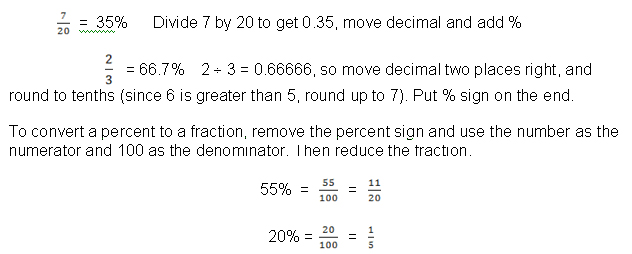Terms – Percentage, Base, Rate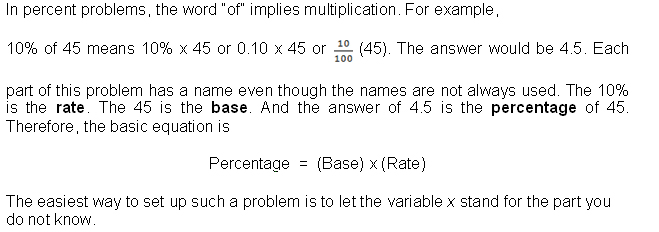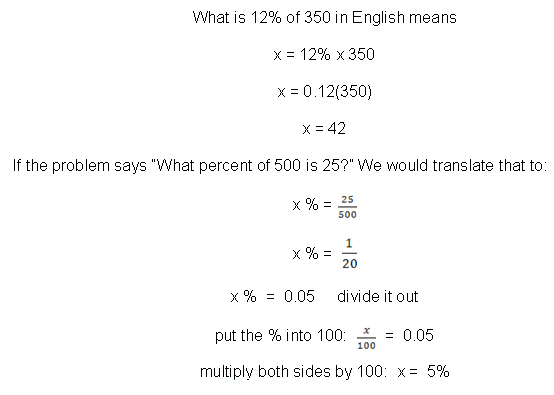Uses of Percent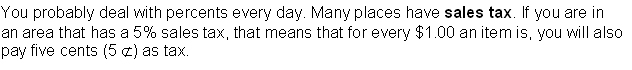If an item is \$32.99, then you will pay 5% more than that with the tax added. First you figure out how much the tax is by taking 5% of \$32.99:

0.05 x 32.99 = 1.6495

Remember that you are dealing with money, so you must round that off to the nearest penny, making it \$1.65. Then you must add that to the \$32.99 in order to know how much you will be paying: \$32.99 + \$1.65 = \$34.64. That is the final price with the sales tax.

Another way to figure it would have been to think about the price as being 100% and the sales tax as 5%, so the total price you pay would be 105%. You could then multiply the original price by 105%:

105% x 32.99 = 1.05(32.99) = 34.6395  =  \$34.64

If you work at a retail store, you may be asked to do markups. This is when you take the wholesale price and increase it by a certain percentage to get the retail price at the store where you work. This increase in price pays your salary and the other expenses of operating the store (rent, lights, heat, etc.).

A sweater may cost \$15 wholesale, but your store makes a profit of 65% on it. Therefore, it must be marked up by 65% to get the retail price.

65% x \$15  =  0.65(15)  =  \$9.75

Now add that to the \$15:  \$9.75  +  \$15  =  \$24.75

The markup is the \$9.75 and the retail price is the \$24.75.

Or, you could look at this as 165% x \$15 which would give you \$24.75 in a single step.

Many stores have markdowns or discounts called sales. This works the opposite of markups and sales tax in that the percentage is subtracted from the original price instead of added to it.

Let's say that same sweater is put on sale for 30% off. That means that you need to find 30% of its retail price and subtract that from its retail price.

30% x \$24.75  =  0.30(24.75)  =  \$7.425 or \$7.43

\$24.75  -  \$7.43  =  \$17.32

In this case, you would subtract the 30% from 100% to do this in a single step:

(100% - 30%) x \$24.75  =  70% x \$24.75  =  0.7 (24.75)  = \$17.325  = \$17.33

It will depend upon how the cash register (which is a computer) is programmed as to whether you are charged \$17.32 or \$17.33, but you can figure out the cost to within a penny this way.

A commission is another place where percents are used. Sales commissions are paid to sales people based on the price of the item they have sold. In some industries, like insurance, it is paid instead of a salary, In many industries, it is a motivator to sell more and is paid in addition to the normal salary.

If a real estate agent makes a 7% commission on a \$175,000 house he sells, he makes

Percentage  =  7% x \$175,000  =  .07 (175,000)  =  \$12,250

Another place that everyone uses percentage is in figuring out a tip. Tips are given to people who serve us – wait staff at a restaurant, a dog groomer, a bartender, a taxicab driver, a valet, etc. Most tips are either 15% or 20%. If you are paying for a meal and the waiter brings the bill and will pick up the bill, you can simply pay the bill plus the tip to him. However, if the waiter brings the bill and you will pay it at a cash register, you should leave the tip on the table and then go pay the bill.

An easy way to figure out a tip without using a calculator: Round the bill to the nearest dollar or half dollar, then move the decimal point one place left to find out 10% of the bill. If you are tipping 20%, double that. If you are tipping 15%, estimate half and add it to the 10%.

If your bill is \$35.95, round it to \$36. Move the decimal point one place left to get \$3.60. That is 10%. Since 2 x 36 is 72, you would tip \$7.20 for a 20% tip.

Half of \$3.60 would be \$1.80 since ½ of 36 is 18. To tip 15%, add \$1.80 to \$3.60 (to estimate, round to \$1.50 and \$4) and tip \$5.40 – your estimate of \$5.50 is close enough to use.

Interest is the biggest use of percentage in everyday life. When you invest money, you make interest – the interest is paid to you. This happens if you have a savings account or you purchase interest-earning bonds or Treasury Bills (TBs) or Certificates of Deposit (CDs).

However, if you borrow money like taking out a loan for a car, boat, or house, you pay interest. And if you use a charge card and do not pay off the charges when they are due, you will be charged interest.

If your loan is for a very short period of time or is a personal loan from a family member, you may pay simple interest. If it is with a bank or financial institution, you will probably pay compound interest. Simple interest is calculated on the entire amount of money (called the principal) once and then the amount is divided by the number of payments and added to each payment. Compound or compounded interest is figured on the principal, then after the first payment, it is calculated on the remainder of the principal and after the next payment it is figured again on the remaining principal and so forth.

To figure interest, you must know the amount of money (principal), the time period for which it was borrowed (time) and the interest rate that is being charged or paid. The formula is:

Interest  =  Principal x Rate x Time

If \$500 is borrowed for 2 years at a 12% interest rate:

Interest  =  \$500 x 12% x 2

Interest  =  (500)(0.12)(2)  =  \$120

The amount owed at the end would be \$500 + \$120 or \$620.

Calculating Compound Interest.

Compound interest is calculated on the principal plus accumulated interest. The amount to be repaid is calculated using the following formula:

A = P( 1 + i )n

For example, you receive 10% interest on a \$1,000 investment in the first year. You reinvested that money back into your original investment. In the second year, you would get 10% interest on the \$1,000 *plus* the \$100 you reinvested. Over the years, compound interest will make you much more money than simple interest because you are reinvesting whatever interest you make. Let's review this in the following example:

A = P( 1 + i )n

A is the final total including the principal.

P is the principal amount (what you originally invested).

i is the rate of interest per year.

n is the number of years invested. Remember n is an exponent.

Example:

Let's say that you have \$2,500.00 to invest for 5 years at a rate of 7% compound interest.

A = 2500 (1 + 0.07)5 = \$3,506.38

You can see that your \$2,500.00 is now worth \$3,506.38 after 5 years at 7% interest compounded annually.